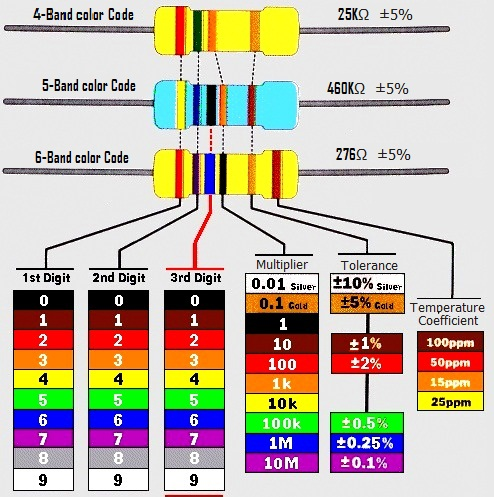# Calculating Resistance is easy## How to Calculate Resistor Value?

Calculating Resistance is easy and simple, yes you can observe the Resistance value of Resistor by using color band over it.

Different types of resistors available in market, for handling resistor we need to know the color code. Resistors may have 4-band color code, 5-band color code or 6-band color code here the reference illustration show the different color code and method of calculating the resistor value.

Before calculating the resistor value hold the resistor in right direction (tolerance band that is Gold or silver to your right hand side and then digit color bands to your left hand side)

Resistor With 4-band color Code = example (RED, GREEN, ORANGE, GOLD)

If you are looking at 4-band color code resistor means the first two color band show the 1st and 2nd digits of resistor value Hence (2 5) then third color band show multiplier (103 or 1000 or 1K) finally the last band shows tolerance (± 5 %)

Hence,

2 5 x 1000 with ± 5 % = 25K &Omega ± 5 %

Now a day’s SMD resistors are mostly used in development boards, by using numbers we can calculate the resistance value of SMD resistor.

In this following link you can find the color code and SMD number of given Resistance Value. http://resisto.rs/